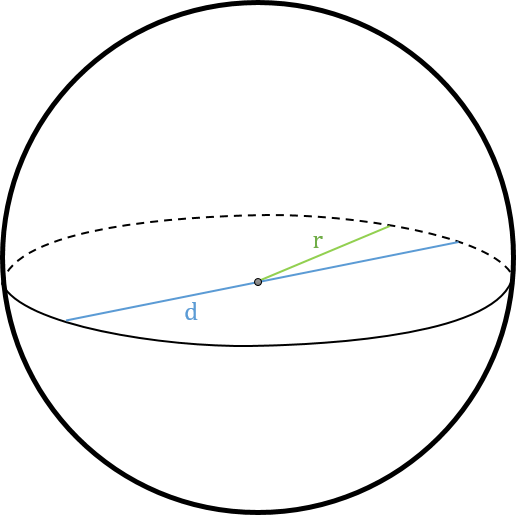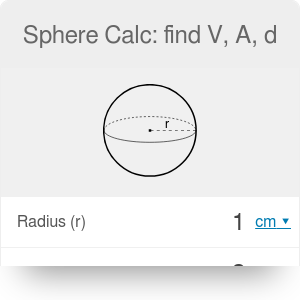in
Diameter (d)
in
Surface area (A)
in²
Volume (v)
cu in
Surface to volume ratio (A / V)
1/
in

# Sphere Calc: find V, A, d

By Dominik Czernia, PhD candidate

Sphere calc is an advanced tool that finds the volume, area and diameter of a sphere. Enter one of your selected quantities to calculate other parameters or read the article below to learn about all the equations we have used. The notation is as follows:• r - radius of a sphere,
• V - volume of a sphere (sphere calc: find V),
• A - area of a sphere (sphere calc: find A),
• d - diameter of a sphere (sphere calc: find d),
• A / V - surface to volume ratio of a sphere.

A sphere is defined as a set of points in three-dimensional space, that are all at the same distance from a given point, which is called the center. Sphere is widely used in physics to model different objects, like spherical capacitors or atoms of a gas. You should also check out our spherical coordinates calculator and see how you can use a sphere to describe the position of any given point in a 3D space.

## Sphere calc: find V

The volume of a sphere V is the space enclosed by a sphere, for example, the space that a substance (solid, liquid or gas) can occupy. Its value is expressed in cubic units of length, e.g., cubic meters `m³` or cubic feet `cu ft`. Try our volume conversion to learn how to convert between different volume units. The volume of a sphere can be found with the following equations:

1. With given radius: `V = 4/3 * π * r³`,
2. With given diameter: `V = 1/6 * π * d³`,
3. With given area: `V = √(A³ / (36 * π))`.

## Sphere calc: find A

The surface area of a sphere A is a measure of the total area that the surface of a sphere occupies. Its value is expressed in squared units of length, e.g., square meters `m²` or square feet `ft²`. Check out area conversion calculator to learn how to convert between different area units! The surface area of a sphere can be found with the following equations:

1. With given radius: `A = 4 * π * r²`,
2. With given diameter: `A = π * d²`,
3. With given volume: `A = ³√(36 * π * V²)`.

## Sphere calc: find d

The diameter of a sphere d is the longest straight line through a sphere, connecting two points of a sphere and passing through its center. The diameter is twice the radius. Diameter, just like radius, is expressed in units of length, e.g., meters `m` or feet `ft`. With our length conversion calculator you can quickly convert between different units of length. The diameter of a sphere can be found with the following equations:

1. With given radius: `d = 2 * r`,
2. With given diameter: `d = √(A / π)`,
3. With given volume: `d = ³√(6 * V / π)`.

## Surface to volume ratio

The interesting fact is that the sphere encloses the largest volume among all other closed surfaces with a given surface area. In other words, the surface to volume ratio `A / V` of a sphere is relatively high compared to other figures. You can easily find an explicit formula for the surface to volume ratio, knowing that the area is `A = 4 * π * r²` and the volume is `V = 4/3 * π * r³`:

`A / V = (4 * π * r²)/(4/3 * π * r³) = 3 / r`

or, if we know that radius is half of diameter `r = d / 2`, then

`A / V = 6 / d`

You can estimate this quantity with our sphere calc too!

Dominik Czernia, PhD candidate

## Get the widget!

Sphere Calc: find V, A, d can be embedded on your website to enrich the content you wrote and make it easier for your visitors to understand your message.

It is free, awesome and will keep people coming back!